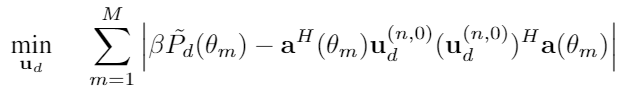Hi! everyone.
I’m new here today.
Kindly i need some help to solve my cvx optimization problem.

cvx_begin sdp
variable U1(n) complex
variable a1(1)
s1 = 0;
for i=1:length(theta)
s1_tmp = abs(-a1*Pd(i) + square_abs(U1’*vec(:,i)))
s1 = s1 + s1_tmp;
end
minimize(s1)
subject to
norm(U1,‘fro’) <= 1
a1 >= 0
cvx_end

When i run this code, i got the error code below:

Disciplined convex programming error:
Illegal operation: abs( {convex} ).

I guess s1_tmp = abs(-a1*Pd(i) + square_abs(U1’*vec(:,i))) term is not supported by cvx.
I prove that the objective function is convex since this is a function of absolute function.

Is there anything cvx expression to perform this?Thank you for reading my question.

I don’t believe there is a rule: “function is convex since this is a function of absolute function”. The absolute value of a convex function is not necessarily convex, and CVX does not allow it.

Do you have a proper proof that your objective function is convex? I will presume it is non-convex until proven otherwise.